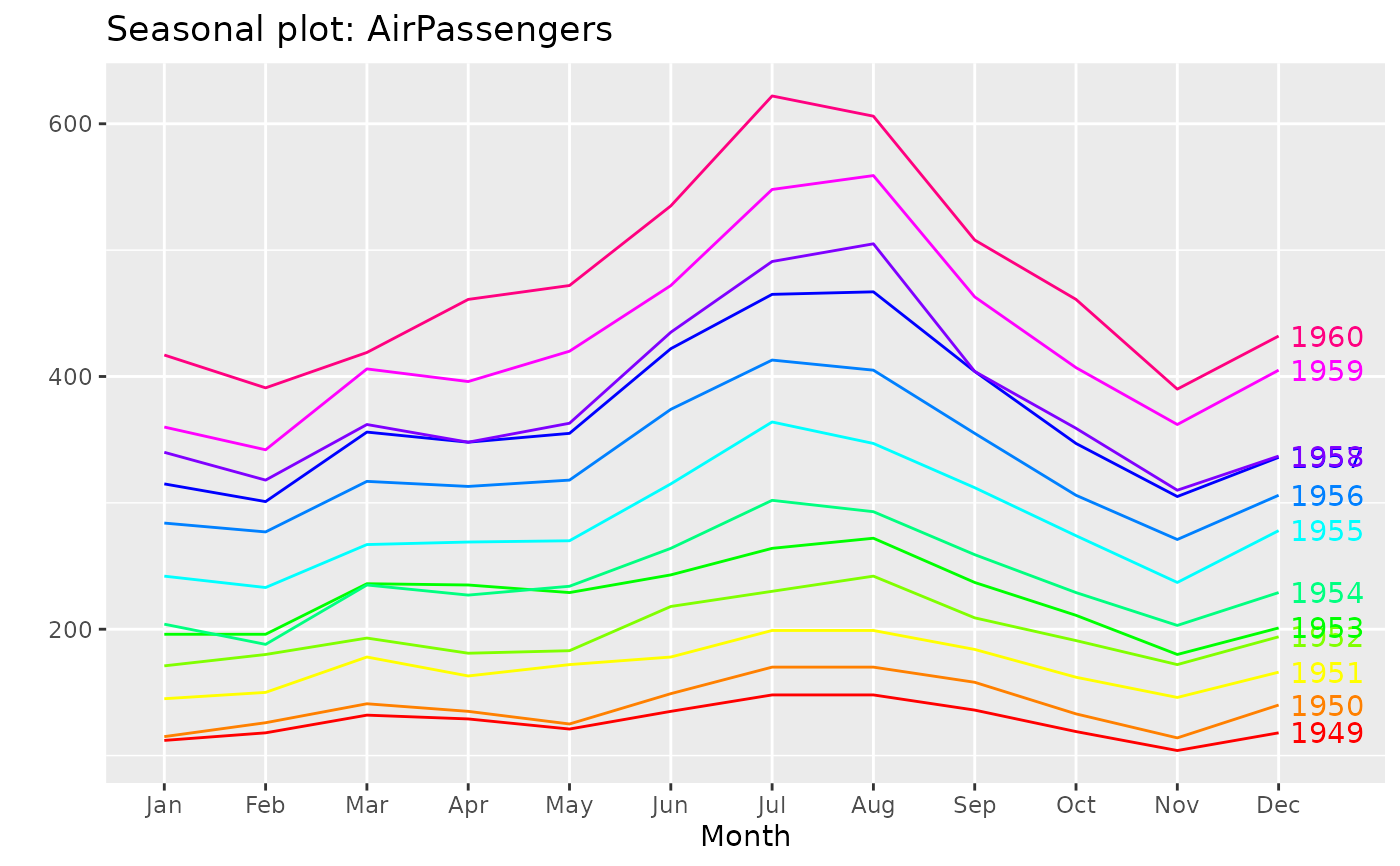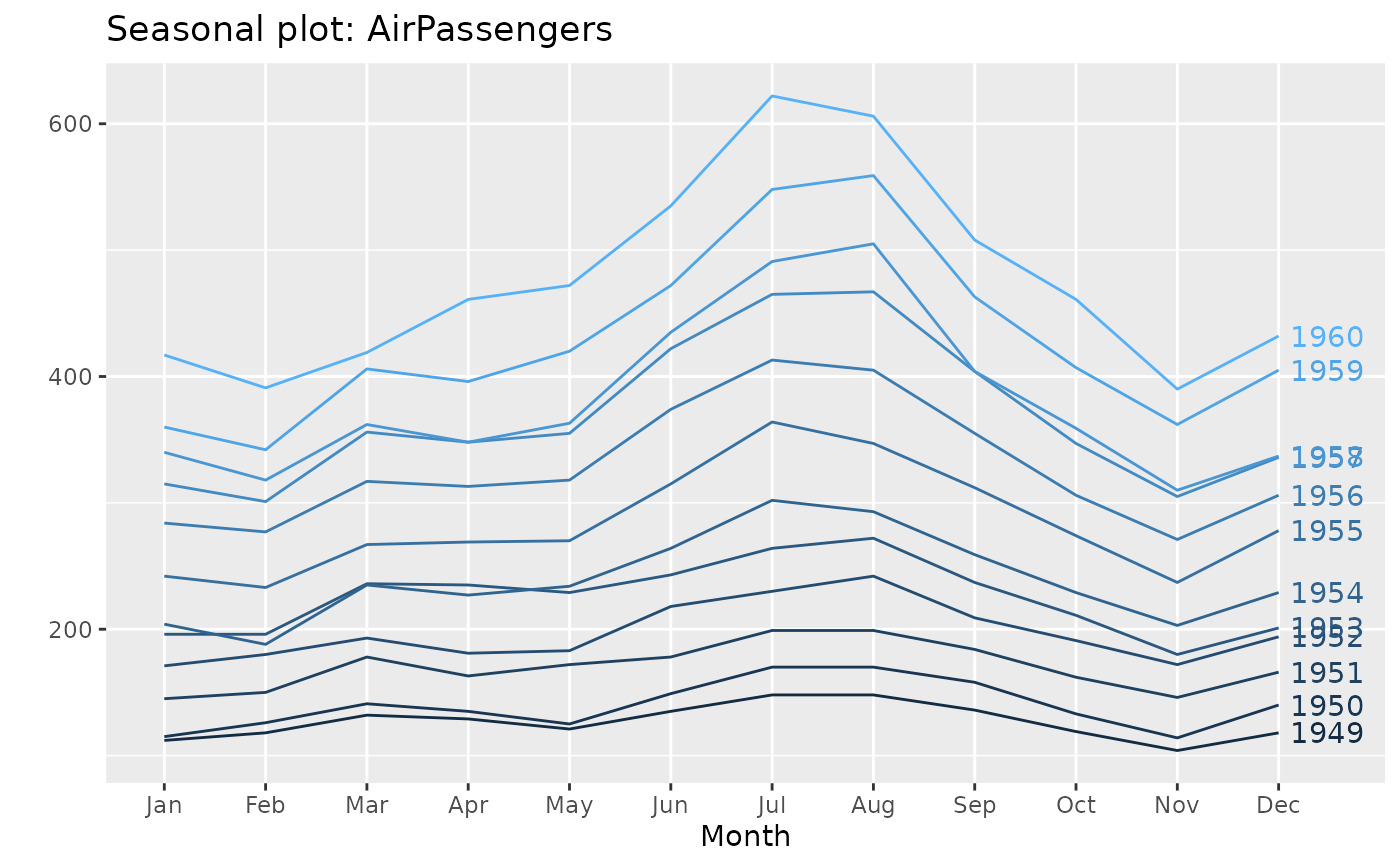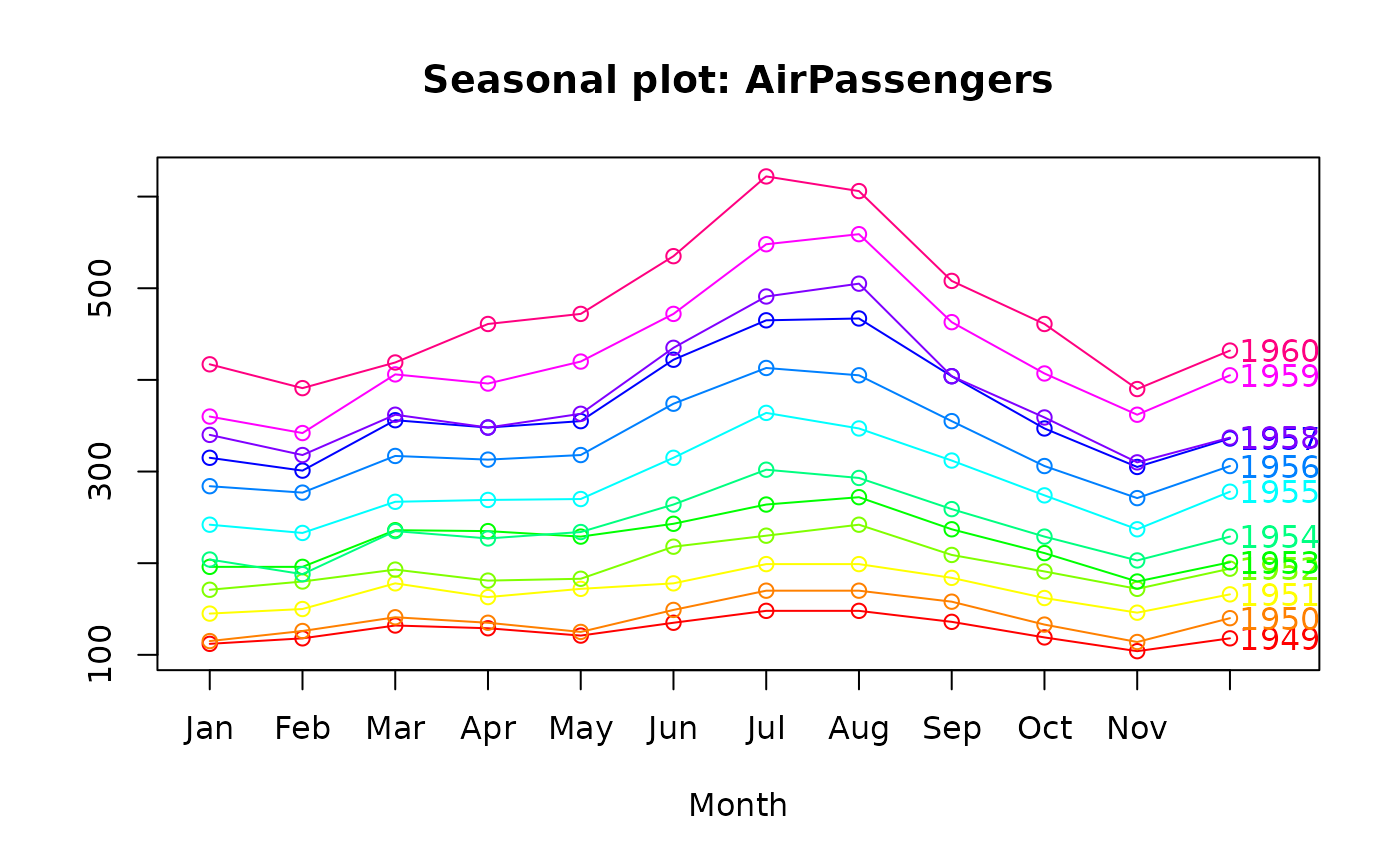Plots a seasonal plot as described in Hyndman and Athanasopoulos (2014, chapter 2). This is like a time plot except that the data are plotted against the seasons in separate years.

ggseasonplot(
x,
season.labels = NULL,
year.labels = FALSE,
year.labels.left = FALSE,
type = NULL,
col = NULL,
continuous = FALSE,
polar = FALSE,
labelgap = 0.04,
...
)

seasonplot(
x,
s,
season.labels = NULL,
year.labels = FALSE,
year.labels.left = FALSE,
type = "o",
main,
xlab = NULL,
ylab = "",
col = 1,
labelgap = 0.1,
...
)

## Arguments

x a numeric vector or time series of class ts. Labels for each season in the "year" Logical flag indicating whether labels for each year of data should be plotted on the right. Logical flag indicating whether labels for each year of data should be plotted on the left. plot type (as for plot). Not yet supported for ggseasonplot. Colour Should the colour scheme for years be continuous or discrete? Plot the graph on seasonal coordinates Distance between year labels and plotted lines additional arguments to plot. seasonal frequency of x Main title. X-axis label. Y-axis label.

## Value

None.

Hyndman and Athanasopoulos (2018) Forecasting: principles and practice, 2nd edition, OTexts: Melbourne, Australia. https://otexts.com/fpp2/

monthplot

## Author

Rob J Hyndman & Mitchell O'Hara-Wild

## Examples

ggseasonplot(AirPassengers, col=rainbow(12), year.labels=TRUE)ggseasonplot(AirPassengers, year.labels=TRUE, continuous=TRUE)seasonplot(AirPassengers, col=rainbow(12), year.labels=TRUE)